### Home > CAAC > Chapter 12 > Lesson 12.2.2 > Problem12-49

12-49.
1. Add, subtract, multiply, or divide the expressions below. Leave your answer as simplified as possible. Homework Help ✎

1.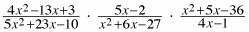2.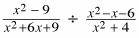3.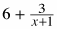4.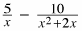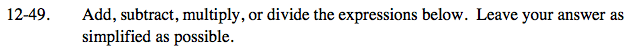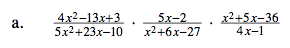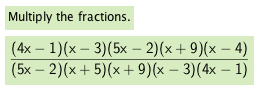Look for 1s.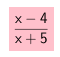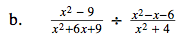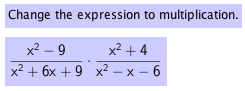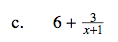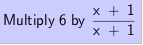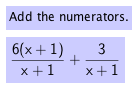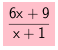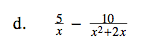Factor the denominator of the term on the right to help you find a common denominator